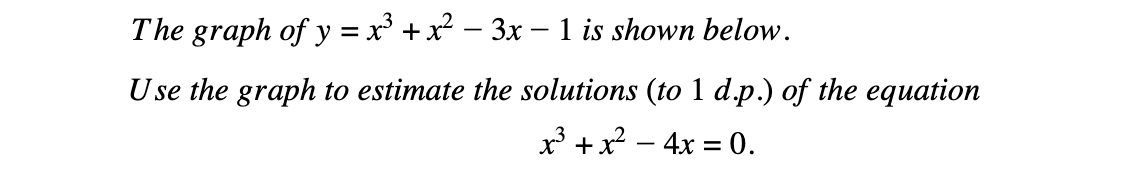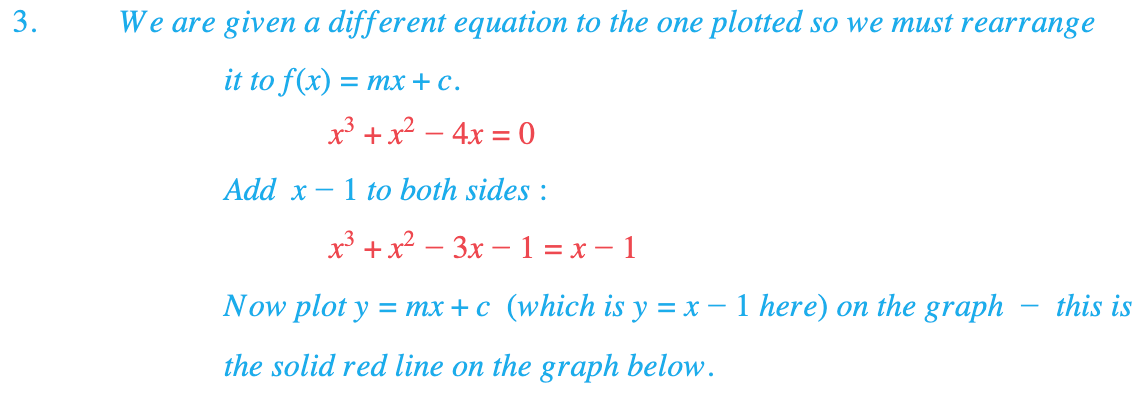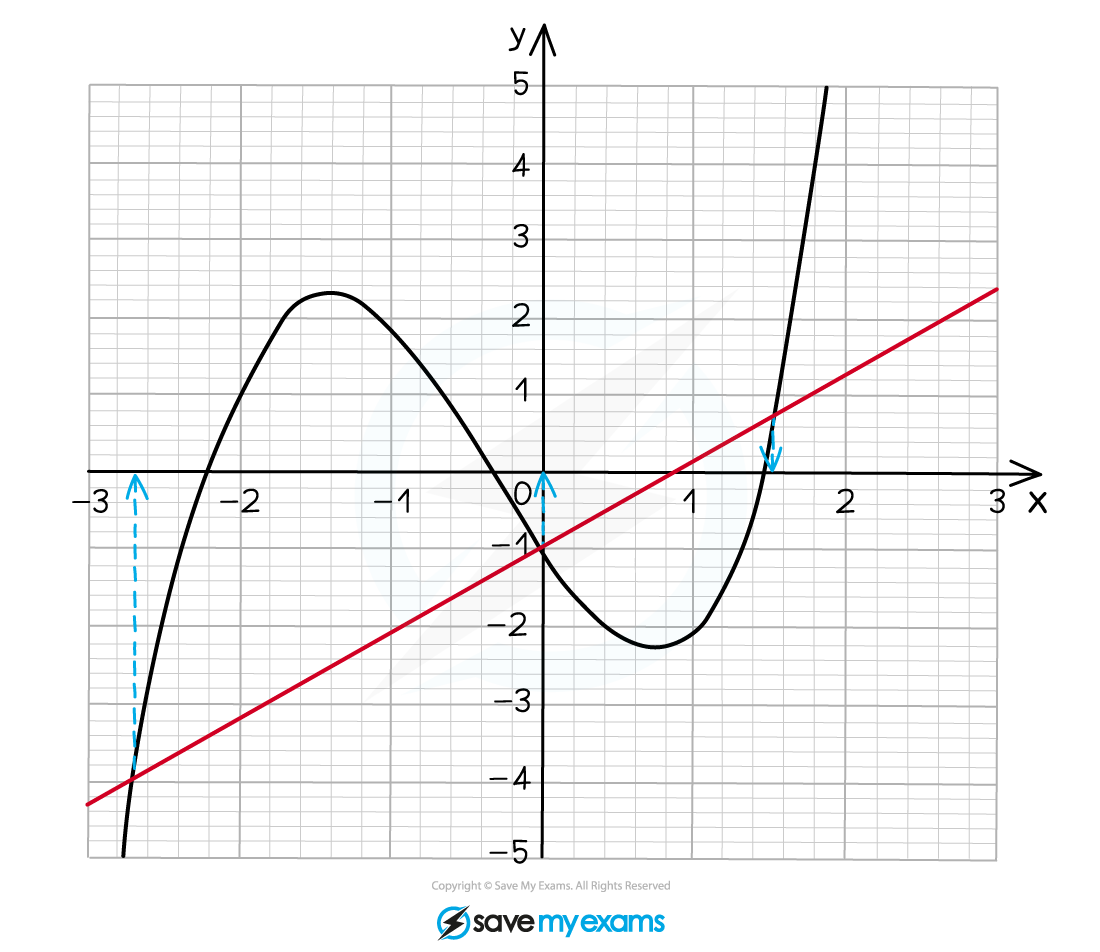# Edexcel IGCSE Maths 复习笔记 3.10.1 Solving Equations using Graphs

Edexcel IGCSE Maths 复习笔记 3.10.1 Solving Equations using Graphs

#### How do we use graphs to solve equations?

• Solutions are always read off the x-axis
• Solutions of f(x) = 0 are where the graph of y = f(x) cuts the x-axis
• If given g(x)=0 instead (Q: “by drawing a suitable straight line”) then:
• Rearrange into f(x) = mx + c and draw the line y = mx + c
• Solutions are the x-coordinates of where the line crosses the curve

Note that solutions may also be called roots

#### Worked Example#### Worked Example